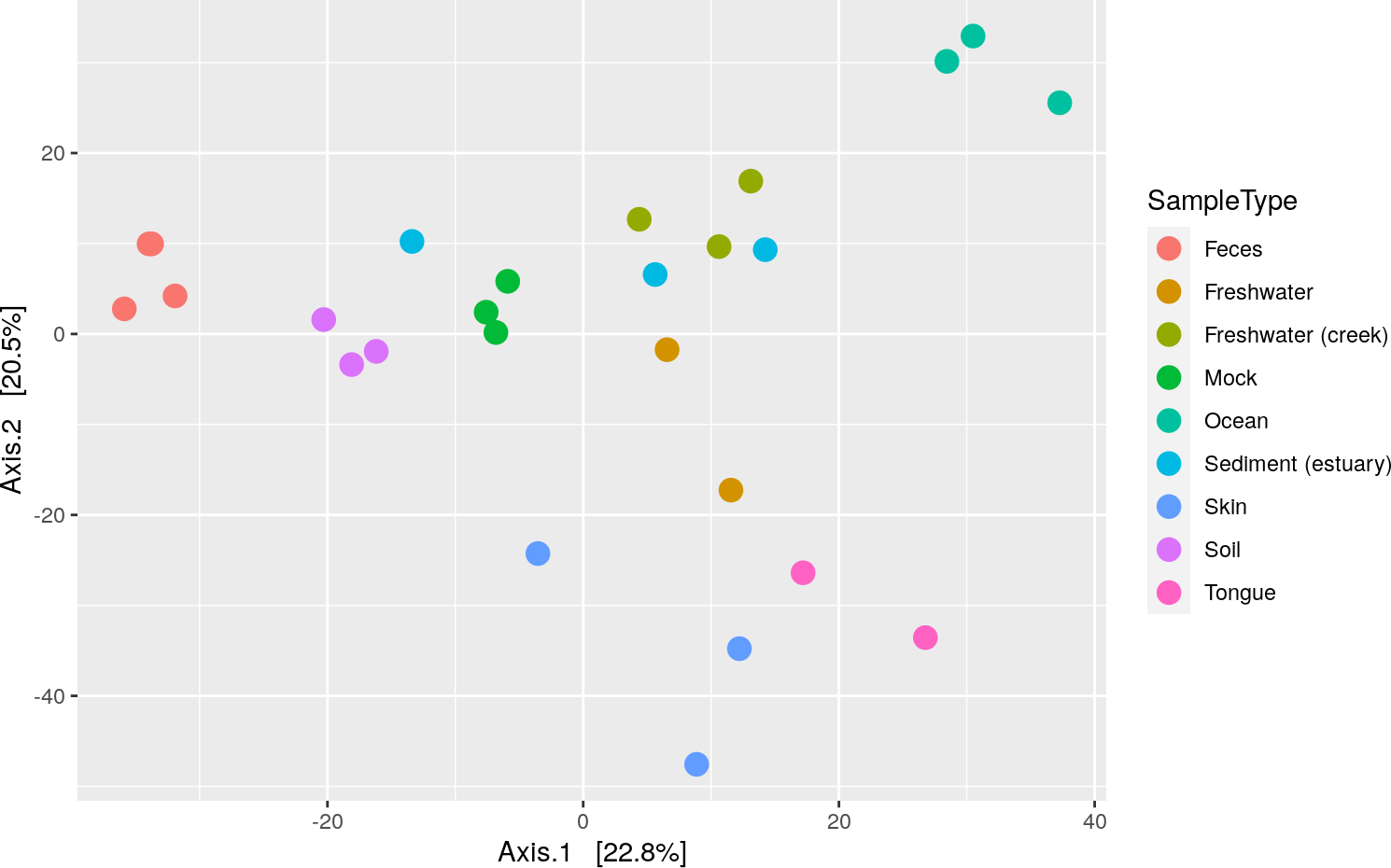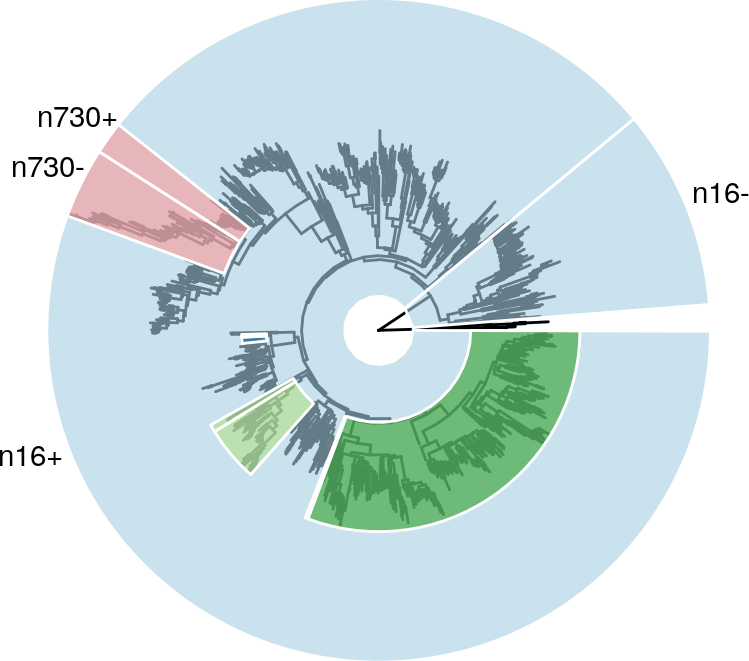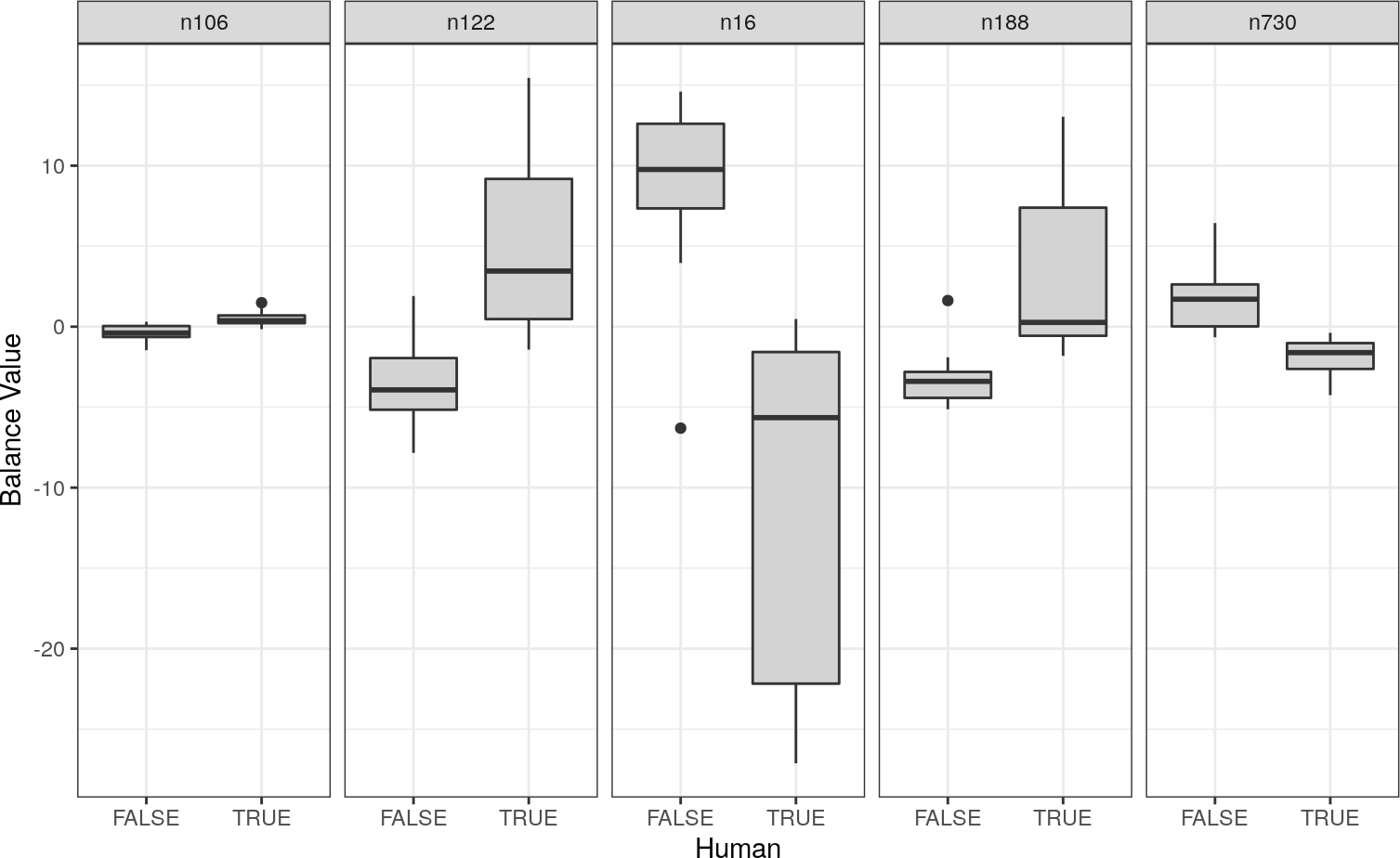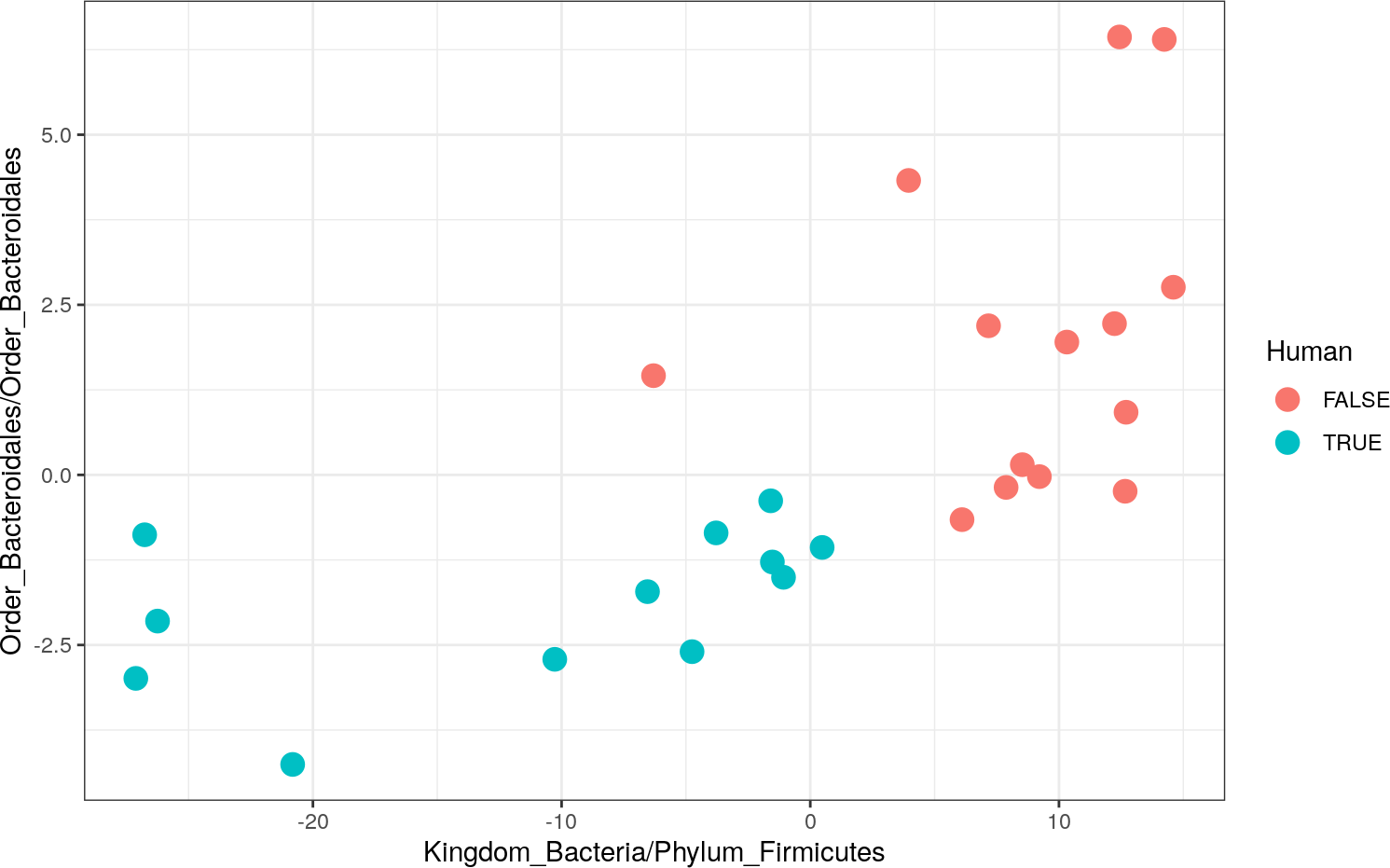# 1 Introduction

PhILR is short for “Phylogenetic Isometric Log-Ratio Transform” (Silverman et al. 2017). This package provides functions for the analysis of compositional data (e.g., data representing proportions of different variables/parts). Specifically this package allows analysis of compositional data where the parts can be related through a phylogenetic tree (as is common in microbiota survey data) and makes available the Isometric Log Ratio transform built from the phylogenetic tree and utilizing a weighted reference measure (Egozcue and Pawlowsky-Glahn 2016).

# 2 Overview of PhILR Analysis

The goal of PhILR is to transform compositional data into an orthogonal unconstrained space (real space) with phylogenetic / evolutionary interpretation while preserving all information contained in the original composition. Unlike in the original compositional space, in the transformed real space, standard statistical tools may be applied. For a given set of samples consisting of measurements of taxa, we transform data into a new space of samples and orthonormal coordinates termed ‘balances’. Each balance is associated with a single internal node of a phylogenetic tree with the taxa as leaves. The balance represents the log-ratio of the geometric mean abundance of the two groups of taxa that descend from the given internal node. More details on this method can be found in Silverman et al. (2017) (Link).

Here we will demonstrate PhILR analysis using the Global Patterns dataset that was originally published by Caporaso et al. (2011). This dataset is provided with the phyloseq package (McMurdie and Holmes 2013) and our analysis follows, in part, that of the authors GitHub Tutorial.

library(philr); packageVersion("philr")
##  '1.14.0'
library(phyloseq); packageVersion("phyloseq")
##  '1.32.0'
library(ape); packageVersion("ape")
##  '5.3'
library(ggplot2); packageVersion("ggplot2")
##  '3.3.0'
data(GlobalPatterns)

## 3.1 Filter Extremely Low-Abundance OTUs

Taxa that were not seen with more than 3 counts in at least 20% of samples are filtered. Subsequently, those witha coefficient of variation ≤ 3 are filtered. These steps follow those of (McMurdie and Holmes 2013). Finally we add a pseudocount of 1 to the remaining OTUs to avoid calculating log-ratios involving zeros. Alternatively other replacement methods (multiplicative replacement etc…) may be used instead if desired; the subsequent taxa weighting procedure we will describe complements a variety of zero replacement methods.

GP <-  filter_taxa(GlobalPatterns, function(x) sum(x > 3) > (0.2*length(x)), TRUE)
GP <-  filter_taxa(GP, function(x) sd(x)/mean(x) > 3.0, TRUE)
GP <- transform_sample_counts(GP, function(x) x+1)

With these two commands we have removed the filtered taxa from the OTU table, pruned the phylogenetic tree, and subset the taxa table. Here is the result of those filtering steps

## phyloseq-class experiment-level object
## otu_table()   OTU Table:         [ 1248 taxa and 26 samples ]
## sample_data() Sample Data:       [ 26 samples by 7 sample variables ]
## tax_table()   Taxonomy Table:    [ 1248 taxa by 7 taxonomic ranks ]
## phy_tree()    Phylogenetic Tree: [ 1248 tips and 1247 internal nodes ]

## 3.2 Process Phylogenetic Tree

Next we check that the tree is rooted and binary (all multichotomies have been resolved).

is.rooted(phy_tree(GP)) # Is the tree Rooted?
##  TRUE
is.binary.tree(phy_tree(GP)) # All multichotomies resolved?
##  TRUE

Note that if the tree is not binary, the function multi2di from the ape package can be used to replace multichotomies with a series of dichotomies with one (or several) branch(es) of zero length .

Once this is done, we name the internal nodes of the tree so they are easier to work with. We prefix the node number with n and thus the root is named n1.

phy_tree(GP) <- makeNodeLabel(phy_tree(GP), method="number", prefix='n')

We note that the tree is already rooted with Archea as the outgroup and no multichotomies are present. This uses the function name.balance from the philr package. This function uses a simple voting scheme to find a consensus naming for the two clades that descend from a given balance. Specifically for a balance named x/y, x refers to the consensus name of the clade in the numerator of the log-ratio and y refers to the denominator.

name.balance(phy_tree(GP), tax_table(GP), 'n1')
##  "Kingdom_Archaea/Kingdom_Bacteria"

## 3.3 Investigate Dataset Components

Finally we transpose the OTU table (philr uses the conventions of the compositions package for compositional data analysis in R, taxa are columns, samples are rows). Then we will take a look at part of the dataset in more detail

otu.table <- t(otu_table(GP))
tree <- phy_tree(GP)
tax <- tax_table(GP)

otu.table[1:2,1:2] # OTU Table
## OTU Table:          [2 taxa and 2 samples]
##                      taxa are columns
##     540305 108964
## CL3      1      1
## CC1      1      2
tree # Phylogenetic Tree
##
## Phylogenetic tree with 1248 tips and 1247 internal nodes.
##
## Tip labels:
##  540305, 108964, 175045, 546313, 54107, 71074, ...
## Node labels:
##  n1, n2, n3, n4, n5, n6, ...
##
## Rooted; includes branch lengths.
head(metadata,2) # Metadata
##     X.SampleID  Primer Final_Barcode Barcode_truncated_plus_T
## CL3        CL3 ILBC_01        AACGCA                   TGCGTT
## CC1        CC1 ILBC_02        AACTCG                   CGAGTT
##     Barcode_full_length SampleType                              Description
## CL3         CTAGCGTGCGT       Soil Calhoun South Carolina Pine soil, pH 4.9
## CC1         CATCGACGAGT       Soil Cedar Creek Minnesota, grassland, pH 6.1
head(tax,2) # taxonomy table
## Taxonomy Table:     [2 taxa by 7 taxonomic ranks]:
##        Kingdom   Phylum          Class            Order
## 540305 "Archaea" "Crenarchaeota" "Thaumarchaeota" "Cenarchaeales"
## 108964 "Archaea" "Crenarchaeota" "Thaumarchaeota" "Cenarchaeales"
##        Family           Genus            Species
## 540305 "Cenarchaeaceae" NA               NA
## 108964 "Cenarchaeaceae" "Nitrosopumilus" "pIVWA5"

# 4 Transform Data using PhILR

The function philr::philr() implements a user friendly wrapper for the key steps in the philr transform.

1. Convert the phylogenetic tree to its sequential binary partition (SBP) representation using the function philr::phylo2sbp()
2. Calculate the weighting of the taxa (aka parts) or use the user specified weights
3. Built the contrast matrix from the SBP and taxa weights using the function philr::buildilrBasep()
4. Convert OTU table to relative abundance (using philr::miniclo()) and ‘shift’ dataset using the weightings (Egozcue and Pawlowsky-Glahn 2016) using the function philr::shiftp().
5. Transform the data to PhILR space using the function philr::ilrp()
6. (Optional) Weight the resulting PhILR space using phylogenetic distance. These weights are either provided by the user or can be calculated by the function philr::calculate.blw().

Note: The preprocessed OTU table should be passed to the function philr::philr() before it is closed (normalized) to relative abundances, as some of the preset weightings of the taxa use the original count data to down weight low abundance taxa.

Here we will use the same weightings as we used in the main paper.

gp.philr <- philr(otu.table, tree,
part.weights='enorm.x.gm.counts',
ilr.weights='blw.sqrt')
## Building Sequential Binary Partition from Tree...
## Building Contrast Matrix...
## Transforming the Data...
## Calculating ILR Weights...
gp.philr[1:5,1:5]
##                 n1         n2         n3         n4          n5
## CL3     -1.3638521  1.9756259  2.6111996 -3.3174292  0.08335109
## CC1     -0.9441168  3.9054807  2.9804522 -4.7771598 -0.05334306
## SV1      5.8436901  5.9067782  6.7315081 -8.8020849  0.08335109
## M31Fcsw -3.9010427 -0.1816618 -0.5432099  0.1705271  0.08335109
## M11Fcsw -5.4554073  0.5398249 -0.5647474  0.5551616 -0.02389182

Now the transformed data is represented in terms of balances and since each balance is associated with a single internal node of the tree, we denote the balances using the same names we assigned to the internal nodes (e.g., n1).

# 5 Ordination in PhILR Space

Euclidean distance in PhILR space can be used for ordination analysis. We do this ordination using tools from the phyloseq package.

gp.dist <- dist(gp.philr, method="euclidean")
gp.pcoa <- ordinate(GP, 'PCoA', distance=gp.dist)
plot_ordination(GP, gp.pcoa, color='SampleType') + geom_point(size=4)# 6 Identify Balances that Distinguish Human/Non-Human

More than just ordination analysis, PhILR provides an entire coordinate system in which standard multivariate tools can be used. Here we will make use of sparse logistic regression (from the glmnet pacakge) to identify a small number of balances that best distinguish human from non-human samples.

First we will make a new variable distinguishing human/non-human

sample_data(GP)$human <- factor(get_variable(GP, "SampleType") %in% c("Feces", "Mock", "Skin", "Tongue")) Now we will fit a sparse logistic regression model (logistic regression with $$l_1$$ penalty) library(glmnet); packageVersion('glmnet') ##  '3.0.2' glmmod <- glmnet(gp.philr, sample_data(GP)$human, alpha=1, family="binomial")

We will use a hard-threshold for the $$l_1$$ penalty of $$\lambda = 0.2526$$ which we choose so that the resulting number of non-zero coefficients is $$\approx 5$$ (for easy of visualization in this tutorial).

top.coords <- as.matrix(coefficients(glmmod, s=0.2526))
top.coords <- rownames(top.coords)[which(top.coords != 0)]
(top.coords <- top.coords[2:length(top.coords)]) # remove the intercept as a coordinate
##  "n16"  "n106" "n122" "n188" "n730"

# 7 Name Balances

To find the taxonomic labels that correspond to these balances we can use the function philr::name.balance(). This funciton uses a simple voting scheme to name the two descendent clades of a given balance separately. For a given clade, the taxonomy table is subset to only contain taxa from that clade. Starting at the finest taxonomic rank (e.g., species) the subset taxonomy table is checked to see if any label (e.g., species name) represents ≥ threshold (default 95%) of the table entries at that taxonomic rank. If no consensus identifier is found, the table is checked at the next-most specific taxonomic rank (etc…).

tc.names <- sapply(top.coords, function(x) name.balance(tree, tax, x))
tc.names
##                                           n16
##          "Kingdom_Bacteria/Phylum_Firmicutes"
##                                          n106
## "Order_Actinomycetales/Order_Actinomycetales"
##                                          n122
##       "Kingdom_Bacteria/Phylum_Cyanobacteria"
##                                          n188
##   "Genus_Campylobacter/Phylum_Proteobacteria"
##                                          n730
##     "Order_Bacteroidales/Order_Bacteroidales"

We can also get more information on what goes into the naming by viewing the votes directly.

votes <- name.balance(tree, tax, 'n730', return.votes = c('up', 'down'))

votes[[c('up.votes', 'Family')]] # Numerator at Family Level
## votes
##                  1
votes[[c('down.votes', 'Family')]] # Denominator at Family Level
## votes
##                 12                  9                 10                  5

# 8 Visualize Results

library(ggtree); packageVersion("ggtree")
##  '2.2.0'
library(dplyr); packageVersion('dplyr')
##  '0.8.5'

Above we found the top 5 coordinates (balances) that distinguish whether a sample is from a human or non-human source. Now using the ggtree (Yu et al. 2016) package we can visualize these balances on the tree using the geom_balance object. To use these functions we need to know the acctual node number (not just the names we have given) of these balances on the tree. To convert between node number and name, we have added the functions philr::name.to.nn() and philr::nn.to.name().
In addition, it is important that we know which clade of the balance is in the numerator (+) and which is in the denominator (-) of the log-ratio. To help us keep track we have created the function philr::annotate_balance() which allows us to easily label these two clades.

tc.nn <- name.to.nn(tree, top.coords)
tc.colors <- c('#a6cee3', '#1f78b4', '#b2df8a', '#33a02c', '#fb9a99')
p <- ggtree(tree, layout='fan') +
geom_balance(node=tc.nn, fill=tc.colors, alpha=0.6) +
geom_balance(node=tc.nn, fill=tc.colors, alpha=0.6) +
geom_balance(node=tc.nn, fill=tc.colors, alpha=0.6) +
geom_balance(node=tc.nn, fill=tc.colors, alpha=0.6) +
geom_balance(node=tc.nn, fill=tc.colors, alpha=0.6)
p <- annotate_balance(tree, 'n16', p=p, labels = c('n16+', 'n16-'),
offset.text=0.15, bar=FALSE)
annotate_balance(tree, 'n730', p=p, labels = c('n730+', 'n730-'),
offset.text=0.15, bar=FALSE)We can also view the distribution of these 5 balances for human/non-human sources. In order to plot with ggplot2 we first need to convert the PhILR transformed data to long format. We have included a function philr::convert_to_long() for this purpose.

gp.philr.long <- convert_to_long(gp.philr, get_variable(GP, 'human')) %>%
filter(coord %in% top.coords)

ggplot(gp.philr.long, aes(x=labels, y=value)) +
geom_boxplot(fill='lightgrey') +
facet_grid(.~coord, scales='free_x') +
xlab('Human') + ylab('Balance Value') +
theme_bw()# 9 Use Balances for Dimension Reduction

Lets just look at balance n16 vs. balance n730 (the ones we annotated in the above tree).

library(tidyr); packageVersion('tidyr')
##  '1.0.2'
gp.philr.long %>%
rename(Human=labels) %>%
filter(coord %in% c('n16', 'n730')) %>%
theme_bw()